Share

# HC Verma solutions for Class 12 Concepts of Physics Vol. 2 chapter 11 - Thermal and Chemical Effects of Current [Latest edition]

Textbook page

#### Chapters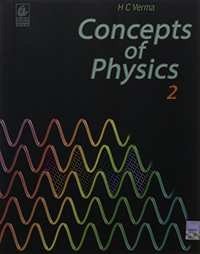## Chapter 11: Thermal and Chemical Effects of Current

### HC Verma solutions for Class 12 Concepts of Physics Vol. 2 Chapter 11 Thermal and Chemical Effects of Current Short Answers [Page 217]

Short Answers | Q 1 | Page 217

If a constant potential difference is applied across a bulb, the current slightly decreases as time passes and then becomes constant. Explain.

Short Answers | Q 2 | Page 217

Two unequal resistances, R1 and R2, are connected across two identical batteries of emf ε and internal resistance r (see the figure). Can the thermal energies developed in R1 and R2 be equal in a given time? If yes, what will be the condition?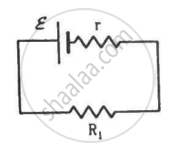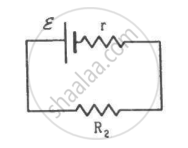Short Answers | Q 3 | Page 217

When a current passes through a resistor, its temperature increases. Is it an adiabatic process?

Short Answers | Q 4 | Page 217

Apply the first law of thermodynamics to a resistor carrying a current i. Identify which of the quantities ∆Q, ∆U and ∆W are zero, positive and negative.

Short Answers | Q 5 | Page 217

Do all thermocouples have a neutral temperature?

Short Answers | Q 6 | Page 217

Is inversion temperature always double the neutral temperature? Does the unit of temperature have an effect in deciding this question?

Short Answers | Q 7 | Page 217

Is neutral temperature always the arithmetic mean of the inversion temperature and the temperature of the cold junction? Does the unit of temperature have an effect in deciding this question?

Short Answers | Q 8 | Page 217

Do the electrodes in an electrolytic cell have fixed polarity like a battery?

Short Answers | Q 9 | Page 217

As temperature increases, the viscosity of liquids decreases considerably. Will this decrease the resistance of an electrolyte as the temperature increases?

### HC Verma solutions for Class 12 Concepts of Physics Vol. 2 Chapter 11 Thermal and Chemical Effects of Current MCQ [Pages 217 - 218]

MCQ | Q 1 | Page 217

Which of the following plots may represent the thermal energy produced in a resistor in a given time as a function of the electric current?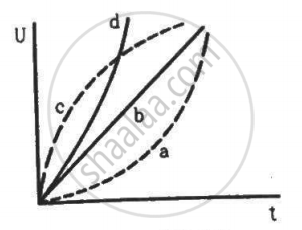MCQ | Q 2 | Page 217

A constant current i is passed through a resistor. Taking the temperature coefficient of resistance into account, indicate which of the plots shown in the figure best represents the rate of production of thermal energy in the resistor.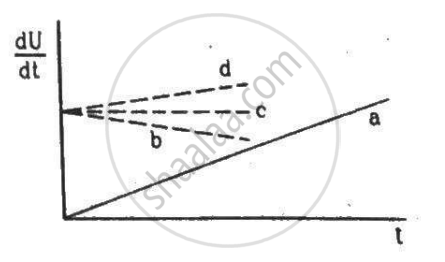MCQ | Q 3 | Page 218

Consider the following statements regarding a thermocouple.
(A) The neutral temperature does not depend on the temperature of the cold junction.
(B) The inversion temperature does not depend on the temperature of the cold junction.

• A and B are correct

• A is correct but B is wrong

• B is correct but A is wrong

• A and B are wrong

MCQ | Q 4 | Page 218

The heat developed in a system is proportional to the current through it.

• It cannot be Thomson heat

• It cannot be Peltier heat

• It cannot be Joule heat

• It can be any of the three heats mentioned above

MCQ | Q 5 | Page 218

Consider the following statements.
(A) Free-electron density is different in different metals.
(B) Free-electron density in a metal depends on temperature.
Seebeck Effect is caused _____________ .

• due to both A and B

• due to A but not due to B

• due to B but not due to A

• neither due to A nor due to B

MCQ | Q 6 | Page 218

Consider the following statements.
(A) Free-electron density is different in different metals.
(B) Free-electron density in a metal depends on temperature.

Peltier Effect is caused _______________ .

• due to both A and B

• due to A but not due to B

• due to B but not due to A

• neither due to A nor due to B

MCQ | Q 7 | Page 218

Consider the following statements.
(A) Free-electron density is different in different metals.
(B) Free-electron density in a metal depends on temperature.

Thomson Effect is caused _______________ .

• due to both A and B

• due to A but not due to B

• due to B but not due to A

• neither due to A nor due to B

MCQ | Q 8 | Page 218

• depends on the amount of the electrolyte

• depends on the current in the electrolyte

• is a universal constant

• depends on the amount of charge passed through the electrolyte

### HC Verma solutions for Class 12 Concepts of Physics Vol. 2 Chapter 11 Thermal and Chemical Effects of Current MCQ [Page 218]

MCQ | Q 1 | Page 218

Two resistors of equal resistances are joined in series and a current is passed through the combination. Neglect any variation in resistance as the temperature changes. In a given time interval,

(a) equal amounts of thermal energy must be produced in the resistors

(b) unequal amounts of thermal energy may be produced

(c) the temperature must rise equally in the resistors

(d) the temperature may rise equally in the resistors

MCQ | Q 2 | Page 218

A copper strip AB and an iron strip AC are joined at A. The junction A is maintained at 0°C and the free ends B and C are maintained at 100°C. There is a potential difference between _______________ .

(a) the two ends of the copper strip
(b) the copper end and the iron end at the junction
(c) the two ends of the iron strip
(d) the free ends B and C

MCQ | Q 3 | Page 218

The constants a and b for the pair silver-lead are 2.50 μV°C−1 and 0.012μV°C−2, respectively. For a silver-lead thermocouple with colder junction at 0°C, ______________ .

(a) there will be no neutral temperature
(b) there will be no inversion temperature
(c) there will not be any thermo-emf even if the junctions are kept at different temperatures
(d) there will be no current in the thermocouple even if the junctions are kept at different temperatures

MCQ | Q 4 | Page 218

An electrolysis experiment is stopped and the battery terminals are reversed.

• The electrolysis will stop

• The rate of liberation of material at the electrodes will increase

• The rate of liberation of material will remain the same

• Heat will be produced at a greater rate

MCQ | Q 5 | Page 218

The electrochemical equivalent of a material depends on _______________ .

• the nature of the material

• the current through the electrolyte containing the material

• the amount of charge passed through the electrolyte

• the amount of this material present in the electrolyte

### HC Verma solutions for Class 12 Concepts of Physics Vol. 2 Chapter 11 Thermal and Chemical Effects of Current [Pages 218 - 219]

Q 1 | Page 218

An electric current of 2.0 A passes through a wire of resistance 25 Ω. How much heat will be developed in 1 minute?

Q 2 | Page 218

A coil of resistance 100 Ω is connected across a battery of emf 6.0 V. Assume that the heat developed in the coil is used to raise its temperature. If the heat capacity of the coil is 4.0 J K−1, how long will it take to raise the temperature of the coil by 15°C?

Q 3 | Page 218

The specification on a heater coil is 250 V, 500 W. Calculate the resistance of the coil. What will be the resistance of a coil of 1000 W to operate at the same voltage?

Q 4 | Page 219

A heater coil is to be constructed with a nichrome wire (ρ = 1.0 × 10−6 Ωm) that can operate at 500 W when connected to a 250 V supply. (a) What would be the resistance of the coil? (b) If the cross-sectional area of the wire is 0.5 mm2, what length of the wire will be needed? (c) If the radius of each turn is 4.0 mm, how many turns will be there in the coil?

Q 5 | Page 219

A bulb with rating 250 V, 100 W is connected to a power supply of 220 V situated 10 m away using a copper wire of area of cross-section 5 mm2. How much power will be consumed by the connecting wires? Resistivity of copper = 1.7 × 10−8 Ωm

Q 6 | Page 219

An electric bulb, when connected across a power supply of 220 V, consumes a power of 60 W. If the supply drops to 180 V, what will be the power consumed? If the supply is suddenly increased to 240 V, what will be the power consumed?

Q 7 | Page 219

A servo voltage stabiliser restricts the voltage output to 220 V ± 1%. If an electric bulb rated at 220 V, 100 W is connected to it, what will be the minimum and maximum power consumed by it?

Q 8 | Page 219

An electric bulb marked 220 V, 100 W will get fused if it is made to consume 150 W or more. What voltage fluctuation will the bulb withstand?

Q 9 | Page 219

An immersion heater rated 1000 W, 220 V is used to heat 0.01 m3 of water. Assuming that the power is supplied at 220 V and 60% of the power supplied is used to heat the water, how long will it take to increase the temperature of the water from 15°C to 40°C?

Q 10 | Page 219

An electric kettle used to prepare tea, takes 2 minutes to boil 4 cups of water (1 cup contains 200 cc of water) if the room temperature is 25°C. (a) If the cost of power consumption is Re 1.00 per unit (1 unit = 1000 watt-hour), calculate the cost of boiling 4 cups of water. (b) What will be the corresponding cost if the room temperature drops to 5°C?

Q 11 | Page 219

The coil of an electric bulb takes 40 watts to start glowing. If more than 40 W are supplied, 60% of the extra power is converted into light and the remaining into heat. The bulb consumes 100 W at 220 V. Find the percentage drop in the light intensity at a point if the supply voltage changes from 220 V to 200 V.

Q 12 | Page 219

The 2.0 Ω resistor shown in the figure is dipped into a calorimeter containing water. The heat capacity of the calorimeter together with water is 2000 J K−1. (a) If the circuit is active for 15 minutes, what would be the rise in the temperature of the water? (b) Suppose the 6.0 Ω resistor gets burnt. What would be the rise in the temperature of the water in the next 15 minutes?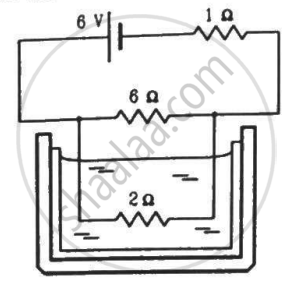Q 13 | Page 219

The temperatures of the junctions of a bismuth-silver thermocouple are maintained at 0°C and 0.001°C. Find the thermo-emf (Seebeck emf) developed. For bismuth-silver, a = − 46 × 10−6 V°C−1 and b = −0.48 × 10−6 V°C−2.

Q 14 | Page 219

Find the thermo-emf developed in a copper-silver thermocouple when the junctions are kept at 0°C and 40°C. Use the data given in the following table.

 Metal with lead (Pb) a mu V"/"^oC b muV"/("^oC) Aluminium -0.47 0.003 Bismuth -43.7 -0.47 Copper 2.76 0.012 Gold 2.90 0.0093 Iron 16.6 -0.030 Nickel 19.1 -0.030 Platinum -1.79 -0.035 Silver 2.50 0.012 Steel 10.8 -0.016
Q 15 | Page 219

Find the neutral temperature and inversion temperature of a copper-iron thermocouple if the reference junction is kept at 0°C. Use the data given in the following table.

 Metal with lead (Pb) a mu V"/"^oC b muV"/("^oC) Aluminium -0.47 0.003 Bismuth -43.7 -0.47 Copper 2.76 0.012 Gold 2.90 0.0093 Iron 16.6 -0.030 Nickel 19.1 -0.030 Platinum -1.79 -0.035 Silver 2.50 0.012 Steel 10.8 -0.016
Q 16 | Page 219

Find the charge required to flow through an electrolyte to liberate one atom of (a) a monovalent material and (b) a divalent material.

Q 17 | Page 219

Find the amount of silver liberated at the cathode if 0.500 A of current is passed through an AgNO3 electrolyte for 1 hour. Atomic weight of silver is 107.9 g mol−1.

Q 18 | Page 219

An electroplating unit plates 3.0 g of silver on a brass plate in 3.0 minutes. Find the current used by the unit. The electrochemical equivalent of silver is 1.12 × 10−6 kg C−1.

Q 19 | Page 219

Find the time required to liberate 1.0 litre of hydrogen at STP in an electrolytic cell by a current of 5.0 A.

Q 20 | Page 219

Two voltameters, one with a solution of silver salt and the other with a trivalent-metal salt, are connected in series and a current of 2 A is maintained for 1.50 hours. It is found  that 1.00 g of the trivalent metal is deposited. (a) What is the atomic weight of the trivalent metal?
(b) How much silver is deposited during this period? Atomic weight of silver is 107.9 g mol−1.

Q 21 | Page 219

A brass plate of surface area 200 cm2 on one side is electroplated with 0.10 mm thick silver layers on both sides using a 15 A current. Find the time taken to do the job. The specific gravity of silver is 10.5 and its atomic weight is 107.9 g/mol−1.

Q 22 | Page 219

The figure shows an electrolyte of AgCl through which a current is passed. It is observed that 2.68 g of silver is deposited in 10 minutes on the cathode. Find the heat developed in the 20 Ω resistor during this period. Atomic weight of silver is 107.9 g/mol−1.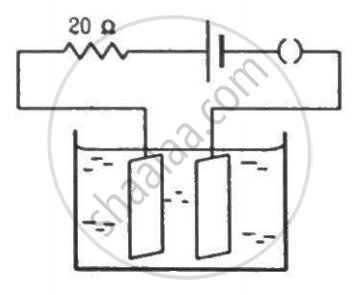Q 23 | Page 219

The potential difference across the terminals of a battery of emf 12 V and internal resistance 2 Ω drops to 10 V when it is connected to a silver voltameter. Find the silver deposited at the cathode in half an hour. Atomic weight of silver is 107.9 g mol−1.

Q 24 | Page 219

A plate of area 10 cm2 is to be electroplated with copper (density 9000 kg m−3) to a thickness of 10 micrometres on both sides, using a cell of 12 V. Calculate the energy spent by the cell in the process of deposition. If this energy is used to heat 100 g of water, calculate the rise in the temperature of the water. ECE of copper = 3 × 10−7 kg C−1and specific heat capacity of water = 4200 J kg−1.

## Chapter 11: Thermal and Chemical Effects of Current## HC Verma solutions for Class 12 Concepts of Physics Vol. 2 chapter 11 - Thermal and Chemical Effects of Current

HC Verma solutions for Class 12 Concepts of Physics Vol. 2 chapter 11 (Thermal and Chemical Effects of Current) include all questions with solution and detail explanation. This will clear students doubts about any question and improve application skills while preparing for board exams. The detailed, step-by-step solutions will help you understand the concepts better and clear your confusions, if any. Shaalaa.com has the CBSE Class 12 Concepts of Physics Vol. 2 solutions in a manner that help students grasp basic concepts better and faster.

Further, we at Shaalaa.com provide such solutions so that students can prepare for written exams. HC Verma textbook solutions can be a core help for self-study and acts as a perfect self-help guidance for students.

Concepts covered in Class 12 Concepts of Physics Vol. 2 chapter 11 Thermal and Chemical Effects of Current are Limitations of Ohm’s Law, Electric Currents in Conductors, Conductivity and Conductance;, Current Density, Delta Star Transformation, Potential Difference and Emf of a Cell, Measurement of Internal Resistance of a Cell, Potentiometer, Metre Bridge, Wheatstone Bridge, Kirchhoff’s Rules, Cells in Series and in Parallel, Cells, Emf, Internal Resistance, Temperature Dependence of Resistance, Combination of Resistors – Series and Parallel, Resistivity of Various Materials, Electrical Resistivity and Conductivity, Electrical Energy and Power, V-I Characteristics (Linear and Non-linear), Ohm’s Law, Drift of Electrons and the Origin of Resistivity, Flow of Electric Charges in a Metallic Conductor, Electric Current.

Using HC Verma Class 12 solutions Thermal and Chemical Effects of Current exercise by students are an easy way to prepare for the exams, as they involve solutions arranged chapter-wise also page wise. The questions involved in HC Verma Solutions are important questions that can be asked in the final exam. Maximum students of CBSE Class 12 prefer HC Verma Textbook Solutions to score more in exam.

Get the free view of chapter 11 Thermal and Chemical Effects of Current Class 12 extra questions for Class 12 Concepts of Physics Vol. 2 and can use Shaalaa.com to keep it handy for your exam preparation